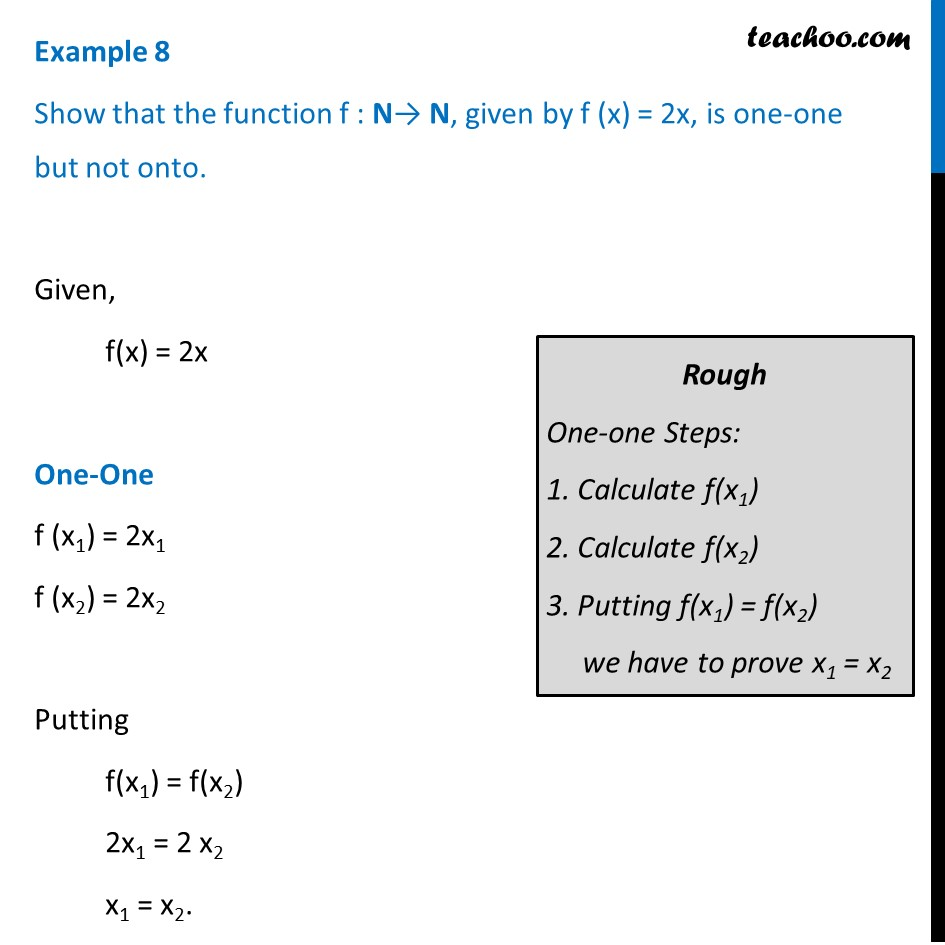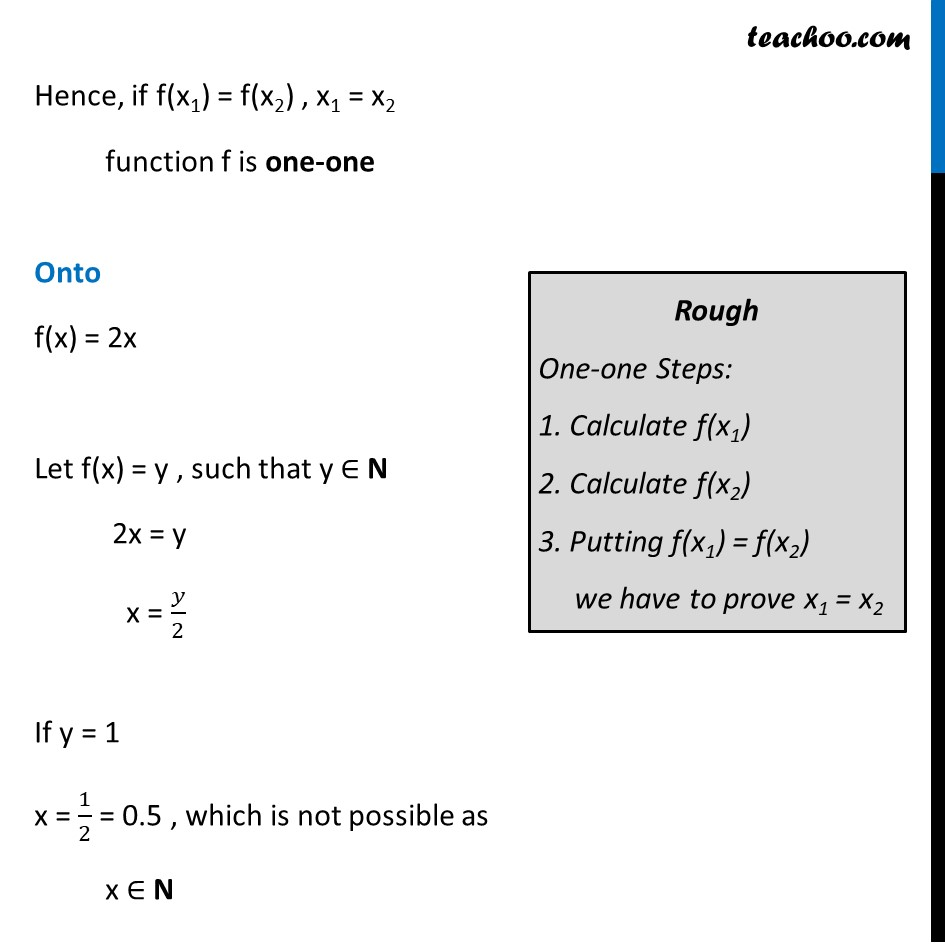Examples

Chapter 1 Class 12 Relation and Functions
Serial order wiseLearn in your speed, with individual attention - Teachoo Maths 1-on-1 Class

### Transcript

Example 8 Show that the function f : N→ N, given by f (x) = 2x, is one-one but not onto. Given, f(x) = 2x One-One f (x1) = 2x1 f (x2) = 2x2 Putting f(x1) = f(x2) 2x1 = 2 x2 x1 = x2. Hence, if f(x1) = f(x2) , x1 = x2 function f is one-one Onto f(x) = 2x Let f(x) = y , such that y ∈ N 2x = y x = 𝑦/2 If y = 1 x = 1/2 = 0.5 , which is not possible as x ∈ N Hence, f is not onto